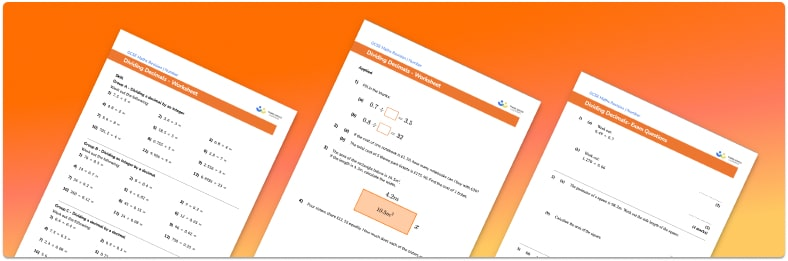# Dividing Decimals Worksheet• Section 1 of the dividing decimals worksheet contains 20+ skills-based dividing decimals questions, in 3 groups to support differentiation
• Section 2 of the decimal division worksheet contains 3 applied decimal division problems with a mix of word problems and deeper problem solving questions
• Section 3 of the dividing decimal numbers worksheet contains 3 foundation and higher level GCSE exam style dividing decimals questions
• Answers and a mark scheme for all dividing decimals questions are provided
• Questions follow variation theory with plenty of opportunities for students to work independently at their own level
• All questions created by fully qualified expert secondary maths teachers
• Suitable for GCSE maths revision for AQA, OCR and Edexcel exam boards

• This field is for validation purposes and should be left unchanged.

You can unsubscribe at any time (each email we send will contain an easy way to unsubscribe). To find out more about how we use your data, see our privacy policy.

### Dividing decimals at a glance

Dividing decimals is an important skill to master, especially when it comes to working with money. To divide a decimal dividend by a divisor which is a whole number, we can use the familiar methods of short or long division. The only difference with the division of decimals is the decimal point, which must be kept in the correct place.

To divide by a decimal number, we make the divisor a whole number by multiplying by an appropriate power of ten (decided by the number of digits after the decimal point). We must also multiply the dividend by the same power of ten. We can then use decimal long division or short division as above. The new calculation will give the same quotient as the original calculation.

For example, if we want to work out 36 divided by 2.4, we notice that the 4 is in the tenths column so to make 2.4 a whole number, we need to multiply by 10. Multiplying both the divisor and the dividend by the same powers of ten, we get 360 divided by 24. We can then use short or long division to solve this, giving us 360 div 24 = 15.

Knowledge of decimal place values of tenths, hundredths, thousandths is useful here.

Looking forward, students can then progress to additional decimals worksheets and other number worksheets, for example a multiplying and dividing decimals worksheet or an order of operations worksheet.For more teaching and learning support on Number our GCSE maths lessons provide step by step support for all GCSE maths concepts.

## Do you have KS4 students who need more focused attention to succeed at GCSE?There will be students in your class who require individual attention to help them succeed in their maths GCSEs. In a class of 30, it’s not always easy to provide.

Help your students feel confident with exam-style questions and the strategies they’ll need to answer them correctly with our dedicated GCSE maths revision programme.

Lessons are selected to provide support where each student needs it most, and specially-trained GCSE maths tutors adapt the pitch and pace of each lesson. This ensures a personalised revision programme that raises grades and boosts confidence.

Find out more